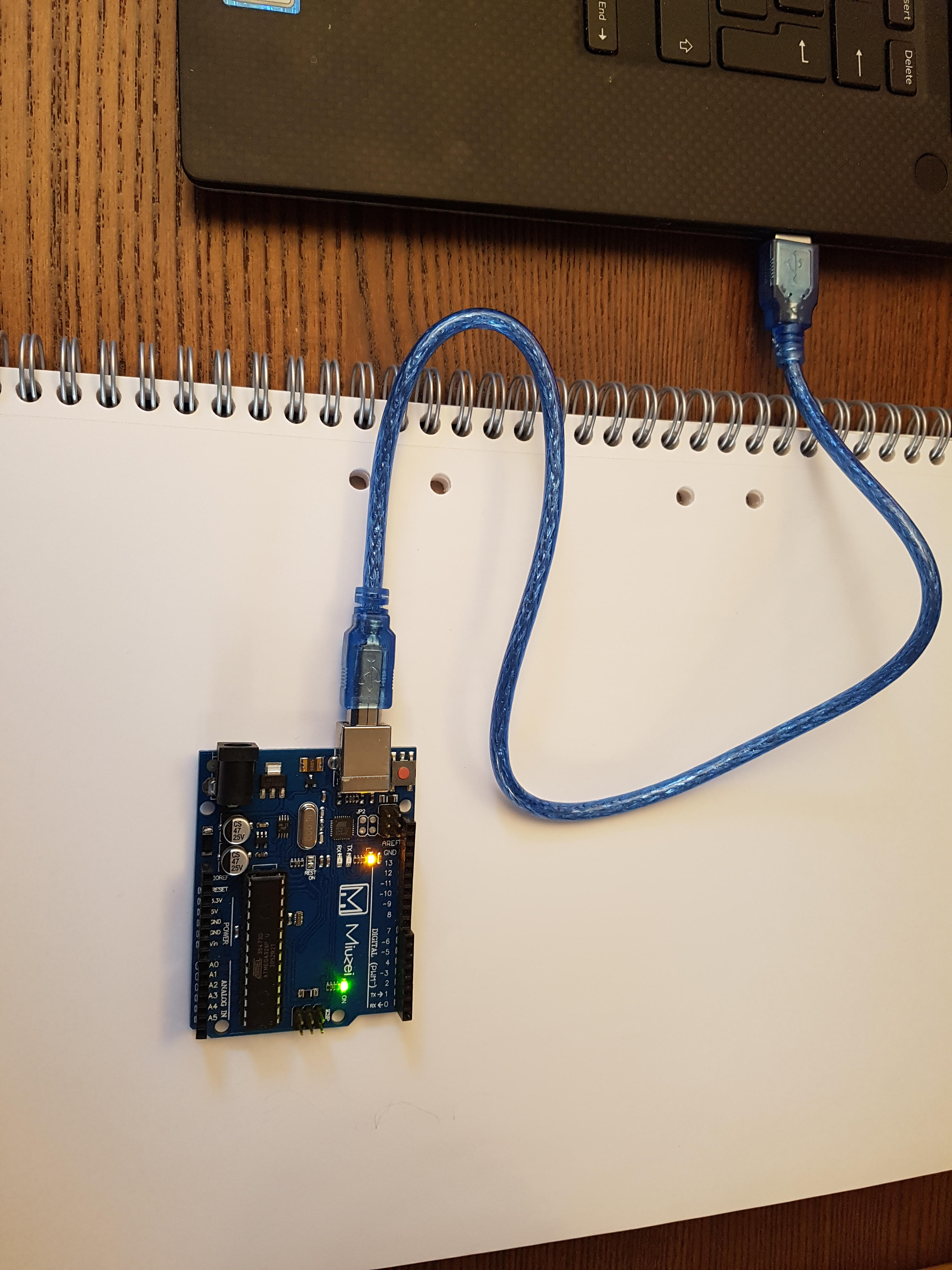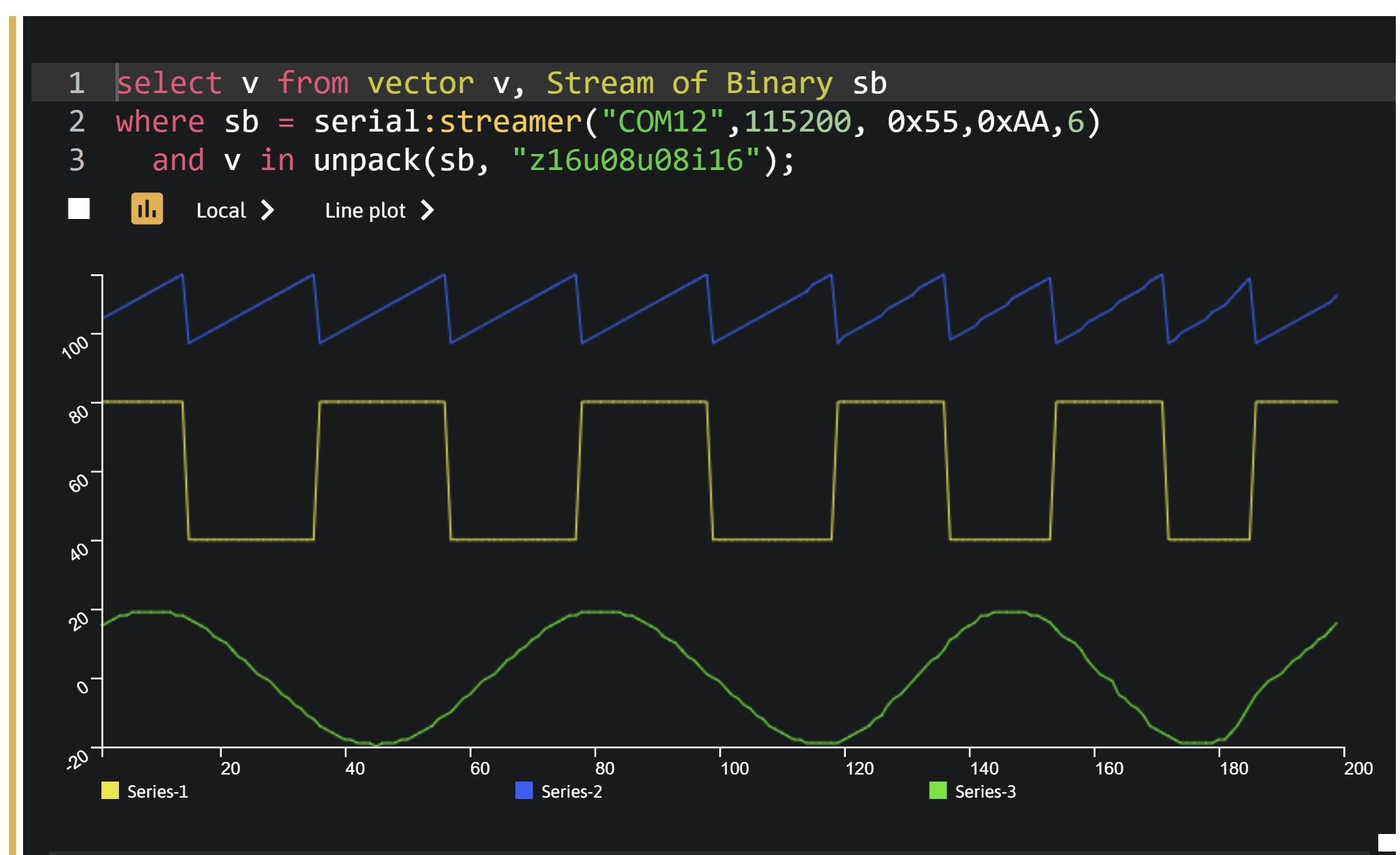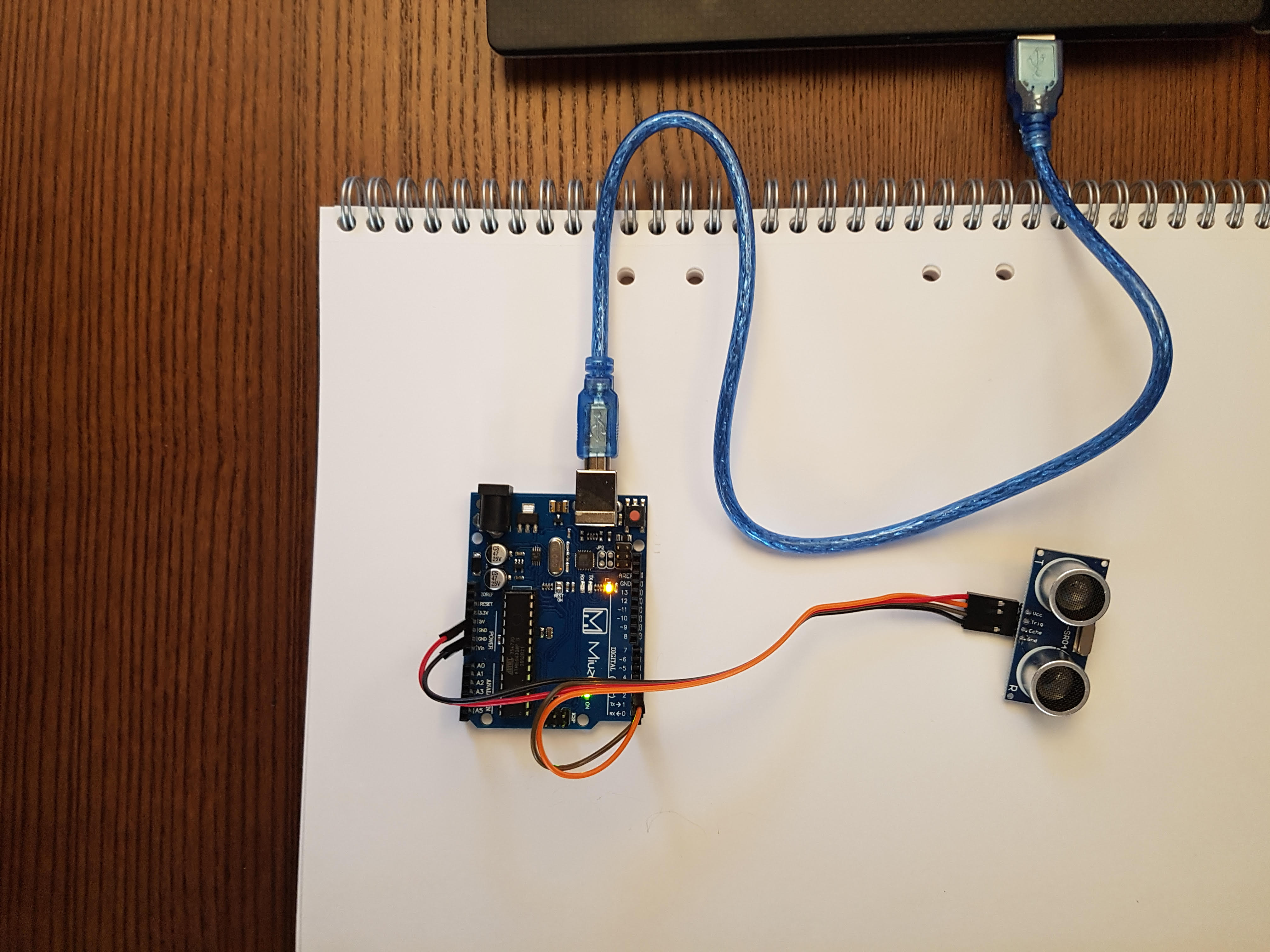# Arduino UNO as sensor

This document will walk you through how to connect an Arduino UNO to provide sensor data to sa.engine.

Hardware:

Prerequisite: You should have basic knowledge of the Arduino IDE. More information can be found here

In the following examples we will use the serial streamer interface. For more information about the serial streamer interface Serial streamer

## Arduino UNO as signal generator​

In the first example we are going to use the Arduino Uno board as a simple signal generator.

Connect the Arduino Uno board to your computerCreate a new sketch in the Arduino IDE. Copy and paste the following code to the sketch and upload it to the Uno board.

const float pi=3.14159;void setup() {  Serial.begin(115200); // open the serial port at 115200 bps:}void loop() {  static uint8_t pkt;  static int i=0;  static int x = 0;  static float y = 0;  static uint8_t square = 60;  static uint8_t height = -1;  y=20*sin(x*pi/180);   //calculate sine, transform deg->rad  pkt = 0x55; //Startbyte  pkt = 0x00; // Not in use  pkt = 0x00; // Not in use  pkt = square+height*20; //square wave  pkt = 97+i; //sawtooth wave  *((int16_t *)&pkt) = (int16_t)(y); //Sine wave  pkt = 0xAA; //Stopbyte  i++;  if(i>20)  {    i=0;    height *=-1;  }  x=x+5;// increase the angle  Serial.write(pkt,8);  // send package  delay(10);}

We will use unpack to extract the integers from the binary data:

mddoc("unpack")
Not connected

To run this code block you must be logged in and your studio instance must be started.

Run the query

select v from vector v, Stream of Binary sbwhere sb = serial:streamer("COM12",115200, 0x55,0xAA,6)  and v in unpack(sb, "z16u08u08i16");
Not connected

To run this code block you must be logged in and your studio instance must be started.

Note

Update the query so it use the correct COM-port (the example use COM12)

If everything is connected correctly, the following should be displayed.Exercise

Modify the Arduino code so the two unused bytes are filled with data. Change the OSQL-query so unpack handles the first 2 bytes as well.

## ARDUINO UNO board with connected sensor​

In the second example we are going to use the Arduino ultrasonic sensor to provide sa.engine with sensor data.

Connect the Arduino distance sensor to the Arduino board.

Connect the four cables from the distance sensor to the Arduino Uno board as follows

• vcc to +5 volt
• gnd to gnd
• trig to digital 3
• echo to digital 2Create a new sketch in the Arduino IDE. Copy and paste the following code to the sketch and upload it to the Uno board.

#define echoPin 2#define triggerPin 3long duration; // variable for the duration of sound wave traveluint16_t  distance; // distance to target in cmvoid setup() {  pinMode(triggerPin, OUTPUT); // Sets the trigPin as an OUTPUT  pinMode(echoPin, INPUT);  Serial.begin(115200);}uint16_t distance_sensor(){  // Clears the trigPin condition  digitalWrite(triggerPin, LOW);  delayMicroseconds(2);  // Sets the triggerPin HIGH (ACTIVE) for 10 microseconds  digitalWrite(triggerPin, HIGH);  delayMicroseconds(10);  digitalWrite(triggerPin, LOW);  // Reads the echoPin, returns the sound wave travel time in microseconds  duration = pulseIn(echoPin, HIGH);  // Calculating the distance  distance = duration * 0.034 / 2; // Speed of sound wave divided by 2 (go and back)  // Displays the distance on the Serial Monitor  return distance;}void loop() {  static uint8_t pkt;   pkt = 0x55; //Startbyte *((uint16_t *)&pkt) = distance_sensor();  pkt = 0xAA; //stopbyte  Serial.write(pkt,4);  delay(10);}

Run the query.

select v from vector v, Stream of Binary sbwhere sb = serial:streamer("COM12",115200, 0x55,0xAA,2)  and v in unpack(sb, "u16");
Not connected

To run this code block you must be logged in and your studio instance must be started.

Note: Update the query so it use the correct COM-port (the example use COM12)

Place an object at different distances in front of the distance sensor and verify that streaming distance changes.